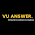# CS201 Assignment 2 Solution 2021 - Introduction to Programming Assignment

Check Here Latest CS201 Fall Assignment

CS201 ASSIGNMENT 2 SOLUTION SPRING 2021

Total Marks: 20

Due Date: 8-June-2021

FREE TO CHANGE ASSIGNMENT WHATSAPP 03162965677

CS201 Assignment Objectives:

In this assignment, the students will learn:

VIEW MORE CORRECT ASSIGNMENT SOLUTIONS

CS101 Assignment 2 Solution 2021

CS302 Assignment 2 Solution 2021

• How to fill a two-dimensional array using required data.

• How to write user-defined functions and pass an array to them as parameters.

• How to use if statement and use while loop.

• How to display an array of elements.

CODE SOLUTION:

// CS201 Assignment 2 Solution Spring 2021

// Provide by VUAnswer.com Free All Solution

#include <iostream>

#include <conio.h>

using namespace std;

int ShowMatrix()

{

int row=2, column=2;

int matrix = { {8, -4} , {-6, 2}  };

cout<<"The matrix is:"<<endl;

for(int i=0; i<row; ++i) {

for(int j=0; j<column; ++j)

cout<<matrix[i][j]<<" ";

cout<<endl;

}

}

int showTranspose ( )

{

int transpose, row=2, column=2, i, j;

int matrix = { {8, -4} , {-6, 2}  };

cout<<endl;

for(i=0; i<row; ++i)

for(j=0; j<column; ++j) {

transpose[j][i] = matrix[i][j];

}

cout<<"The transpose of the matrix is:"<<endl;

for(i=0; i<column; ++i) {

for(j=0; j<row; ++j)

cout<<transpose[i][j]<<" ";

cout<<endl;

}

}

int calculateDeterminant()

{

int  determMatrix, determinant;

int matrix = { {8, -4} , {-6, 2}  };

determinant = ((matrix * matrix) -

(matrix * matrix));

cout << "\nThe Determinant of 2 * 2 Matrix = " << determinant;

}

{

//Calculating co-factors of matrix of order 2x2

C2=A2; C2=-A2; C2=-A2; C2=A2;

//calculating ad-joint of matrix of order 2x2

cout<<"\n\nAdjoint of A is :- \n\n";

}

int main()

{

int choice = 0;

cout<<""<<endl;

cout<<"---Press 1 to display the matrix and its transpose---"<<endl;

cout<<"---Press 2 to find adjoint and determinant of the matrix---"<<endl;

cout<<""<<endl;

cout<<"Press any other key to exit.";

cout<<""<<endl;

// If you want free to change assignment solution

// Whatsapp us 03162965677

cin>>choice;

if (choice ==1)

{

ShowMatrix();

showTranspose ( );

}

else if (choice == 2)

{

calculateDeterminant();

}

else

system("pause");

}

SUCCESS CODE RESULT JUST FOR ACCURACYMake sure you can make some changes to your solution file before submitting copy-paste solution will be marked zero.

If you found any mistake then correct yourself and inform me.

Before submitting an assignment check your assignment requirement file.

If you need some help and question about file and solutions.

CS201 Assignment 2
VU CS201 Assignment 2 solution 2021
Introduction to programming assignment 2 solution 2021

1.1.